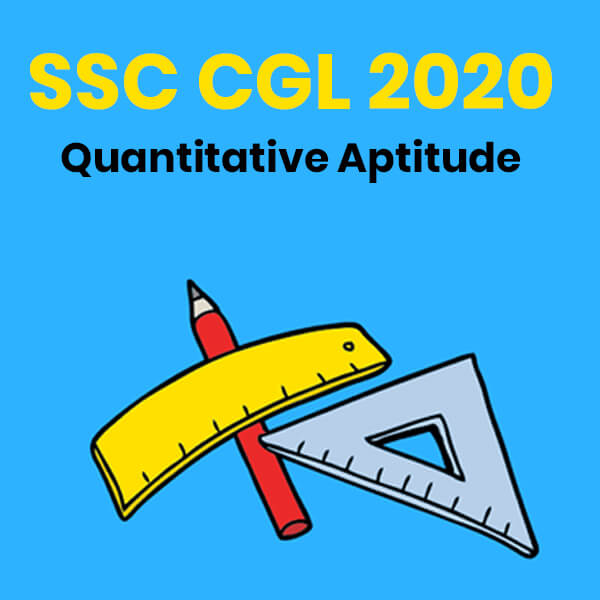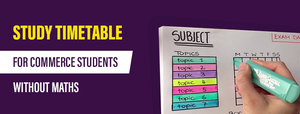Quantitative Aptitude - SSC CGL 2020 - Study24x7New to Study24X7 ?

# Quantitative Aptitude - SSC CGL 2020

Updated on 15 February 2020SSC 2021 Preparation Strategie
Updated on 15 February 2020The aspirants who are within the SSC CGL 2020 age limit must start the SSC CGL 2020 preparation in a systematic manner now as the SSC CGL 2020 exam dates are already out and are only months away. Some of the important topics from the quantitative aptitude syllabus are as follows:Computation of whole number

What is the whole number?

The whole numbers are set of natural numbers including 0.

Set of Whole Number= {0, 1, 2, 3, 4, 5, 6, . …., n)

• Natural numbers are a subset of whole numbers.
• Zero (0) is the smallest whole number.
• The sum of two even whole numbers always gives even the whole number.

Example: 2+4=6

• The sum of two whole odd numbers always gives an even whole number.

Example: 1+3= 4

• The sum of one even and one odd whole number always gives an odd whole number.

Example: 2+ 3= 5

Properties of whole numbers:

If a, b, and c are whole numbers then they will show the following properties on different arithmetic operations:

• Closure Property: Only addition and multiplication operation hold this property.

a + b = n, where n is a whole number.

a * b= m, where m is again a whole number.

• Commutative Property: The subtraction and division operations do not follow commutative property.

a + b= b + a

a * b= b * a

• Associative Property:

The associative property is shown by only addition and multiplication operation.

(a + b) + c= a + (b + c)

(a * b) * c= a * (b * c)

• Distributive property:

The addition and multiplication operations together show distributive property.

a* (b + c)= a * b  + b * c

• Identity element for Addition and Subtraction:

If 0 is added or subtracted in any whole number then you will get the same whole number as a result.

a + 0= a

a – 0= a

• Identity element for Multiplication and Division:

If 1 is multiplied or divided into a whole number then you will get the same whole number in the result.

a * 1= a

a / 1= a

NOTE:

To test the above properties you can put any value of a, b and c from the set of whole numbers.

Decimals, Fractions, and Relationship between Numbers

Decimal: A number that separates itself with a dot (.) called decimal point is known as decimal number.

Representation: 3.14

Here the number 3 is an integer and 14 is the fraction part with a decimal point or dot (.) between them.

Fraction: Fractions are the way of representing the part of numbers in the form of p/q. Here p and q are known as numerator and denominator respectively for which q ≠ 0.

Representation:

p / q= 2/5 is a fraction where p= 2 and q= 5.

Conversion of decimal into fraction:

If you want to convert a decimal number into fraction you need to follow the below steps:

• In the denominator place a 1.
• Count the number of digits after the decimal point.
• Put the same number of zeros after 1 in the denominator and you will get the equivalent fraction.

Example:

3.14= 314/100

All The Best To All the SSC CGL 2020 Aspirants !

Write a comment...Trending ArticlesBy CBSE CLASS 8By CBSE CLASS 10By Examination GuideBy K-12-Commerce
Related Posts